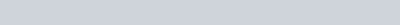# Lecture in Data and Signals | Forouzan

(Last Updated On: March 21, 2020)

### Definition of Terms

• Data must be transformed to electromagnetic signals to be transmitted.
• Data can be analog or digital. Analog data are continuous and take continuous
values. Digital data have discrete states and take discrete values.
• Signals can be analog or digital. Analog signals can have an infinite number of
values in a range; digital signals can have only a limited number of values.
• In data communications, we commonly use periodic analog signals and nonperiodic
digital signals.
• A signal is periodic if it consists of a continuously repeating pattern.
• Frequency and period are the inverse of each other.
• Frequency is the rate of change with respect to time.
• Change in a short span of time means high frequency. Change over a long span of time means low frequency.
• If a signal does not change at all, its frequency is zero. If a signal changes instantaneously, its frequency is infinite.
• Phase describes the position of the waveform relative to time O.
• A time-domain graph plots amplitude as a function of time.
• A frequency-domain graph plots each sine wave’s peak amplitude against its frequency.
• A complete sine wave in the time domain can be represented by one single spike in
the frequency domain.
• A single-frequency sine wave is not useful in data communications; we need to send a composite signal, a signal made of many simple sine waves.
• According to Fourier analysis, any composite signal is a combination of simple sine waves with different frequencies, amplitudes, and phases.
• The spectrum of a signal consists of the sine waves that make up the signal.
• If the composite signal is periodic, the decomposition gives a series of signals with discrete frequencies; if the composite signal is nonperiodic, the decomposition gives a combination of sine waves with continuous frequencies.
• The bandwidth of a composite signal is the difference between the highest and the lowest frequencies contained in that signal.
• Bit rate (number of bits per second) and bit interval (duration of 1 bit) are terms used to describe digital signals.
• A digital signal is a composite analog signal with an infinite bandwidth.
• Bit rate and bandwidth are proportional to each other.
• Baseband transmission of a digital signal that preserves the shape of the digital
signal is possible only if we have a low-pass channel with an infinite or very wide bandwidth.
• Baseband transmission means sending a digital or an analog signal without modulation
using a low-pass channel.
• Broadband transmission means modulating a digital or an analog signal using a band-pass channel.
• If the available channel is a bandpass channel, we cannot send a digital signal
directly to the channel; we need to convert the digital signal to an analog signalbefore transmission.
• For a noiseless channel, the Nyquist bit rate formula defines the theoretical maximum bit rate. For a noisy channel, we need to use the Shannon capacity to find the maximum bit rate.
• The Shannon capacity gives us the upper limit; the Nyquist formula tells us how many signal levels we need.
• Optical signals have very high frequencies. A high frequency means a short wave length because the wave length is inversely proportional to the frequency (λ = v/f), where v is the propagation speed in the media.
• Attenuation is the loss of a signal’s energy due to the resistance of the medium.
• The decibel measures the relative strength of two signals or a signal at two different points.
• Distortion is the alteration of a signal due to the differing propagation speeds of each of the frequencies that make up a signal.
• Noise is the external energy that corrupts a signal.
• The bandwidth-delay product defines the number of bits that can fill the link.
• The wavelength of a frequency is defined as the propagation speed divided by the frequency.
• If a signal does not change at all, its frequency is zero. If a signal changes instantaneously, its frequency is infinite.
• The Shannon capacity gives us the upper limit; the Nyquist formula tells us how many signal levels we need.
• The bandwidth-delay product defines the number of bits that can fill the link.

• Analog
• Digital

• Amplitude
• Frequency
• Phase

• Attenuation
• Distortion
• Noise

### We can evaluate transmission media by

• Throughput – a measure of how fast we can actually send data through a network. An actual measurement of how fast we can send data.
• Propagation speed – depends on the medium and on the frequency of the signal. In a vacuum, light is propagated with a speed of 3 x 108 m/s. It is lower in air and  it is much lower in cable.
• Propagation time – measures the time required for a bit to travel from the source to the destination. The propagation time is calculated by dividing the distance by the propagation
speed.

### In networking, we use the term bandwidth in two contexts.

• The first, bandwidth in hertz, refers to the range of frequencies in a composite signal or the range of frequencies that a channel can pass.
• The second, bandwidth in bits per second, refers to the speed of bit transmission in a channel or link.

### Formulas

• Frequency and Period
•• Number of bits of each Level
•• Decibel
•, dB = 20 log10(V2/V1)

• Noiseless Channel: Nyquist Bit Rate
•• Noisy Channel: Shannon Capacity
•• Signal-to-Noise Ratio (SNR)
• SNR = Average Signla Power/Average Noise Power
SNRdb = 10log10SNR

Note: You can proceed to take the multiple choice exam regarding this topic. Data and Signals – Set 1 MCQs